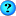View Single Post
rhiegerAuthorized User
 Points: 52, Level: 1Activity: 0%Join Date: Jul 2008
Location: New York, NY, USA.
Posts: 11
Thanks: 0
Thanked 0 Times in 0 PostsPROBLEM WITH CHAPTER 4, EXERCISE 3

I am having a problem getting Exercise 3 from chapter 4 of the book to work. At first, I tried to solve the problem on my own and could not. Finally, I resorted to the code in Appendix A and studied it very carefully to see if I understood it. I am still struggling to grasp its logic, but basically understand it.

I then entered the code from Appendix A, p. 665. With very minor deviation from what appears in the text, here is my code:

CODE FOR fix(fixNumber, decimalPlaces) FUNCTION
=====================================

<script type="text/javascript">

/* fix() Function
================================================== ===================

This function takes input of a number and requested number of decimal
places from a user in two separate prompt boxes.

It then calculates what and how many decimal places to display on
the number entered by the user.

================================================== ====================
*/

function fix(fixNumber, decimalPlaces) {

// Declare and initialize variable to hold the exponential value of
// decimal places for the number entered by the user.

var div = Math.pow(fixNumber, decimalPlaces);

// Declare String object used for searching position of decimal place.

fixNumber = new String(Math.round(fixNumber * div) / div);

// Search new String object for position of decimal place.

if (fixNumber.lastIndexOf(".") == -1) {

fixNumber = fixNumber + "."; // If no decimal places, add only
// decimal point to string.

} // end if

// Determine zeroes required.

var zeroesRequired = decimalPlaces -
( fixNumber.length - fixNumber.lastIndexOf(".") - 1 )

for (; zeroesRequired > 0; zeroesRequired--) {

fixNumber = fixNumber + "0";

} // end for

return fixNumber; // Returns value of fixNumber to calling function.

} // end fix(fixNumber, decimalPlaces)

</script>

CODE FOR SCRIPT WITHIN HTML BODY
============================

<script type="text/javascript">

// Prompt for user to enter a number.

var number1 = prompt("Enter a number with decimal places whose number of decimal places\nyou wish to fix: ", "");
var number2 = prompt("How many decimal places do you want?", "");

// Now print value to browser window.

document.write(number1 + " fixed to " + number2 + " decimal places is: ");
document.write( fix(number1, number2) );

</script>

A sample run of the web page where number1 = 2.10246 and number2 = 3 produced the following output in every browser I tested:

2.10246 fixed to 3 decimal places is: 2.1520223122955024

The expected result, according to the book, is 2.100. No matter what I do so far, I cannot produce this result.

Any insight or suggestions would be greatly appreciated.

Sincerely,

Robert Hieger